# 10 Classification Methods From Scikit Learn We Should Know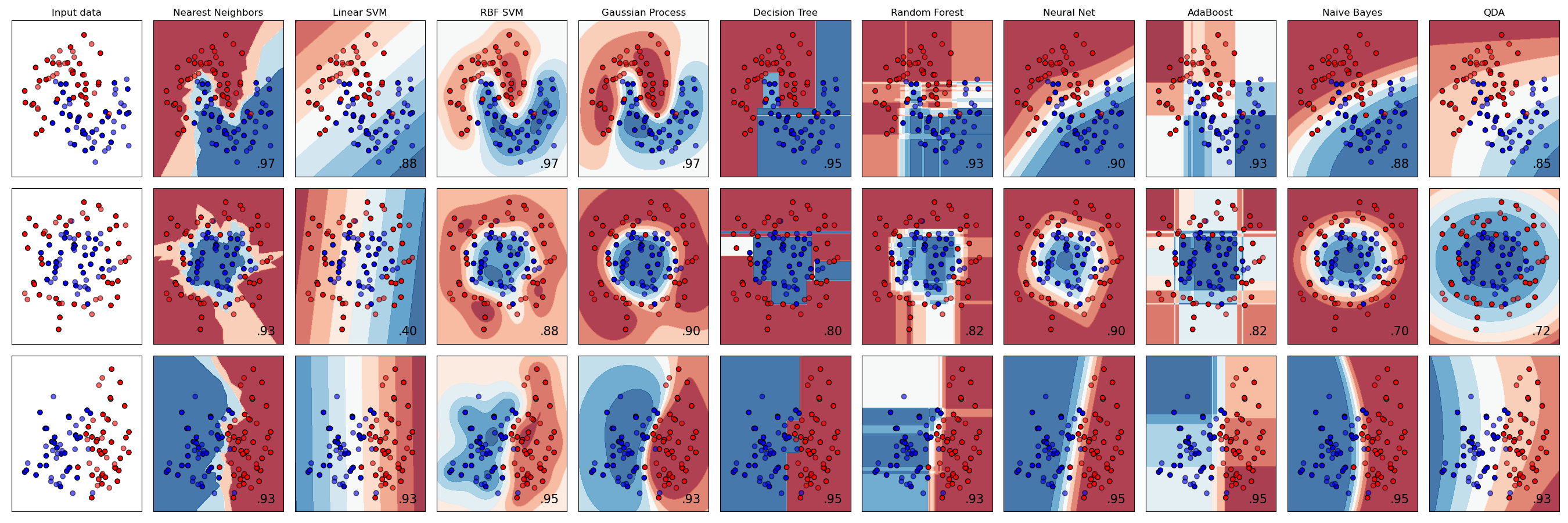Classifier comparison using Scikit Learn

cikit Learn is an open source, Python based very popular machine learning library. It supports various supervised (regression and classification) and unsupervised learning models.

In this blog, we’ll use 10 well known classifiers to classify the Pima Indians Diabetes dataset (download from here and for details, refer here). Instead of going deep into the algorithms or mathematical details, we have limited our discussion on using the Scikit Learn classification methods only. We haven’t included further model optimization/hyper-parameter tuning which can be part of further detailed discussions.

The database contains the following details:

`1. Number of times pregnant2. Plasma glucose concentration a 2 hours in an oral glucose tolerance test3. Diastolic blood pressure (mm Hg)4. Triceps skin fold thickness (mm)5. 2-Hour serum insulin (mu U/ml)6. Body mass index (weight in kg/(height in m)^2)7. Diabetes pedigree function8. Age (years)9. Class variable (0 or 1)`

Let’s load the data and use it as a Spark table…

`df = spark.table ('pima_indians_diabetes')print(f"""There are {df.count()} records in the dataset.""")df.show(5)`

…and convert the Spark DataFrame into a Panda DataFrame:

`import pandas as pddataset = df.toPandas()`

# Data Analysis

## Mean & Standard Deviation

If we want to have a quick look about how the data are distributed around the mean, we can describe the dataset as follows:

`dataset.describe().transpose()`

## Scatter Matrix

These are another important metrics.

Scatter matrix is a set of scatter plots (scatter plot is a mathematical diagram to show the correlation in-between two variable or features) among several feature pairs in a matrix format. This is used to determine if the pair of features are correlated and if the correlation (linear relationship) is positive or negative.

• Positive correlation = if feature 1 increases, feature 2 increases as well.
• Negative correlation = if feature 1 increases, feature 2 decreases.

Scatter matrix is used to verify how independent the input features are and if dimensionality reduction is possible.

`sampled_data = df.drop ("Class").sample(False, 0.8).toPandas()axs = pd.plotting.scatter_matrix(sampled_data, figsize=(10, 10))num_cols = len(sampled_data.columns)for cur_col in range(num_cols):    ax = axs[cur_col, 0]    ax.yaxis.label.set_rotation(0)    ax.yaxis.label.set_ha('right')    ax.set_yticks(())    h = axs[num_cols-1, cur_col]    h.xaxis.label.set_rotation(90)    h.set_xticks(())`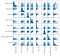The scatter matrix shows, the input features are not correlated.

# Data Preprocessing

## Train & Test Datasets

In the dataset, the last attribute is the dependent variable/label and rest are independent attributes or features. Let’s extract the features & labels:

`X = dataset.iloc[:,:-1].valuesy = dataset.iloc[:, -1].values`

We’ll now split the dataset into random train and test subsets using sklearn library.

`from sklearn.model_selection import train_test_splitX_train, X_test, y_train, y_test = \        train_test_split( \                         X, y, \                         test_size = 0.25, \                         random_state = 0)`

## Standard Scaling

Some algorithms need scaling the features into a same scale while some others (e.g. tree based algorithms) are invariant to it. This process is called Feature Scaling. In this blog we’ll use StandardScaler.

`from sklearn.preprocessing import StandardScalersc = StandardScaler()X_train = sc.fit_transform(X_train)X_test = sc.transform(X_test)`

Now, our dataset is ready to be used by different classifiers.

# 1. Logistic Regression

Logistic Regression is a classification algorithm created based on the logistic function — Sigmoid activation function to convert the outcome into categorical value. This function produces a S-shaped curve which takes any number as input and produces an output in-between 0 and 1 (in case of Binary Logistic Regression).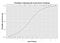Example: Graph of a logistic regression curve showing probability of passing an exam versus hours studying (reference: Wikipedia).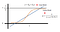Reference: https://www.saedsayad.com/logistic_regression.htm

Logistic regression can be of three types:

1. Binomial / Binary: Dependent variable can have only two possible types, “0” and “1”.
2. Multinomial: Dependent variable can have three or more possible types.
3. Ordinal: Dependent variables that are ordered.

Here, we’ll use only the Binary one to predict the output.

## Implementation

Training the Logistic Regression model (reference: library) on the training set:

`from sklearn.linear_model import LogisticRegressionlr = LogisticRegression(random_state = 0)lr.fit(X_train, y_train)`

Predicting the test set results:

`y_pred_lr = lr.predict(X_test)`

Creating the confusion matrix & calculating accuracy score:

`from sklearn.metrics import confusion_matrix, accuracy_scorecm_lr = confusion_matrix(y_test, y_pred_lr)print(cm_lr)acc_lr = accuracy_score(y_test, y_pred_lr)print (acc_lr)`

## Confusion Matrix

Confusion Matrix is used to evaluate the accuracy of a classification model. The method we used is: sklearn.metrics.confusion_matrix(y_true, y_pred, *, labels=None, sample_weight=None, normalize=None), where y_true is the truth target values and y_pred is the predicted values returned by a classifier.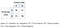Reference: Wikipedia

## Accuracy Score

if the entire set of predicted labels for a sample strictly match with the true set of labels, then the subset accuracy is 1.0; otherwise it is 0.0 — refer: Scikit-Learn

# 2. K-Nearest Neighbours (K-NN)

This model calculates the class membership of the dependent variable by calculating distance with its k nearest neighbors. KNN model is very compute intensive for larger datasets.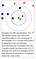Image source: Wikipedia

## Implementation

Training the K-NN model (reference: library) on the training set:

`from sklearn.neighbors import KNeighborsClassifierknn = KNeighborsClassifier(n_neighbors = 5, \                           metric = 'minkowski', p = 2)knn.fit(X_train, y_train)`

Here, we have chosen minkowski as a fast distance metric function.

Predicting the test set results:

`y_pred_knn = knn.predict(X_test)`

Creating the confusion matrix & calculating accuracy score:

`from sklearn.metrics import confusion_matrix, accuracy_scorecm_knn = confusion_matrix(y_test, y_pred_knn)print (cm_knn)acc_knn = accuracy_score(y_test, y_pred_knn)print (acc_knn)`

# 3. SVC (Support Vector Classifier) with Linear Kernel

This model returns a best-fit hyperplane that divides, or categorizes, the input data. This assumes that the data are linearly separable.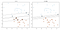Image source: Scikit Learn

## Implementation

Training the SVC model (reference: library) on the training set:

`from sklearn.svm import SVCsvc = SVC(kernel = 'linear', random_state = 0)svc.fit(X_train, y_train)`

Predicting the test set results:

`y_pred_svc = svc.predict(X_test)`

Creating the confusion matrix & calculating accuracy score:

`from sklearn.metrics import confusion_matrix, accuracy_scorecm_svc = confusion_matrix(y_test, y_pred_svc)print (cm_svc)acc_svc = accuracy_score(y_test, y_pred_svc)print (acc_svc)`

# 4. Kernel SVM (Support Vector Machine)

In SVC the kernel function can be any of the following: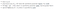Source: scikit-learn

The polynomial, RBF (Radial Basis Function) and sigmoid kernels are especially useful when the data-points are not linearly separable. In this section, we’ll use RBF kernel.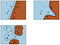Image source: Scikit Learn; using linear, polynomial & RBF kernels respectively.

## Implementation

`from sklearn.svm import SVCsvc_rbf = SVC(kernel = 'rbf', random_state = 0)svc_rbf.fit(X_train, y_train)`

Predicting the test set results:

`y_pred_svc_rbf = svc_rbf.predict(X_test)`

Creating the confusion matrix & calculating accuracy score:

`from sklearn.metrics import confusion_matrix, accuracy_scorecm = confusion_matrix(y_test, y_pred_svc_rbf)print (cm)acc_svc_rbf = accuracy_score(y_test, y_pred_svc_rbf)print (acc_svc_rbf)`

# 5. Naïve Bayes

Naïve Bayes classifiers are a collection of algorithms based on Bayes’ Theorem.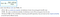Bayes’ Theorem — mathematical formula, source: Wikipedia

All naïve Bayes classifiers assume that the value of a particular feature is independent of the value of the other features, given the class variable. Given, class variable y and dependent feature vector x1 through xn,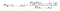Refer the Probabilistic model for further reading.

Few classifiers (in this blog we’ll use Gaussian NB):

## Implementation

Training the NB model (reference: library) on the training set:

`from sklearn.naive_bayes import GaussianNBnb = GaussianNB()nb.fit(X_train, y_train)`

Predicting the test set results:

`y_pred = nb.predict(X_test)`

Creating the confusion matrix & calculating accuracy score:

`from sklearn.metrics import confusion_matrix, accuracy_scorecm = confusion_matrix(y_test, y_pred)print(cm)acc_nb = accuracy_score(y_test, y_pred)print(acc_nb)`

# 6. Decision Tree

A decision tree is a series of if-then-else rules learned from your data for classification or regression tasks. This method commonly used in data mining.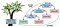Image source: Learning Spark, 2nd Edition, figure 10–9. Decision tree example.

## Implementation

Training the Decision Tree model (reference: library) on the training set:

`from sklearn.tree import DecisionTreeClassifierdt = DecisionTreeClassifier(criterion = 'entropy', random_state = 0)dt.fit(X_train, y_train)`

The criterion parameter selects the function to calculate information gain and the information gain is used to split a node:

• gini
• entropy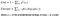Reference: source

Predicting the test set results:

`y_pred = dt.predict(X_test)`

Making the confusion matrix & calculating accuracy score:

`from sklearn.metrics import confusion_matrix, accuracy_scorecm = confusion_matrix(y_test, y_pred)print (cm)acc_dt = accuracy_score(y_test, y_pred)print (acc_dt)`

# 7. Random Forest

Random Forest is an ensemble of Decision Trees by taking various sub-samples of the dataset and uses averaging or majority voting to improve the predictive accuracy. It’s based on the concept that, averaging/voting predictions from different models will be more robust than a prediction of any individual model.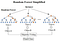Image source: Wikipedia

## Implementation

Training the Random Forest model on the training set:

`from sklearn.ensemble import RandomForestClassifierrf = RandomForestClassifier(max_depth=2, random_state=0)rf.fit(X_train, y_train)`

Few of the parameters (reference library):

• n_estimators: the number of trees in the forest, default=100.
• criterion: the function to measure the quality of a split, either “gini” or “entropy”, default=“gini”.
• max_depth: the maximum depth of the tree.
• max_features: the number of features to consider when looking for the best split — “auto” (sqrt(n_features)) / “sqrt” (sqrt(n_features)) / “log2” (log2(n_features)), default=“auto

Predicting the test set results:

`y_pred_rf = rf.predict(X_test)`

Creating the confusion matrix & calculating accuracy score:

`from sklearn.metrics import confusion_matrix, accuracy_scorecm = confusion_matrix(y_test, y_pred_rf)print (cm)acc_rf = accuracy_score(y_test, y_pred_rf)print (acc_rf)`

# 8. AdaBoost Classifier

AdaBoost (Adaptive Boosting) can be used in conjunction with many other types of learning algorithms to improve performance.

## Implementation

Training the AdaBoost model (reference library) on the training set:

`from sklearn.ensemble import AdaBoostClassifierabc = AdaBoostClassifier()abc.fit(X_train, y_train)`

The algorithm parameter takes either:

• SAMME or,
• SAMME.R (default; this typically converges faster than SAMME)

Predicting the test set results:

`y_pred_abc = abc.predict(X_test)`

Creating the confusion matrix & calculating accuracy score:

`from sklearn.metrics import confusion_matrix, accuracy_scorecm = confusion_matrix(y_test, y_pred_abc)print (cm)acc_abc = accuracy_score(y_test, y_pred_abc)print (acc_abc)`

# 9. Quadratic Discriminant Analysis

A quadratic classifier is statistical classifier that uses a quadratic decision surface to separate measurements of two or more classes of objects or events. It is a more general version of the linear classifier. — Wikipedia

This has no hyperparameters to tune.

## Implementation

Training the quadratic discriminant analysis model (reference library) on the training set:

`from sklearn.discriminant_analysis \     import QuadraticDiscriminantAnalysisqda = QuadraticDiscriminantAnalysis()qda.fit(X_train, y_train)`

Predicting the test set results:

`y_pred_qda = qda.predict(X_test)`

Making the confusion matrix & calculating accuracy score:

`from sklearn.metrics import confusion_matrix, accuracy_scorecm = confusion_matrix(y_test, y_pred_qda)print (cm)acc_qda = accuracy_score(y_test, y_pred_qda)print (acc_qda)`

# 10. MLP Classifier

Multi-Layer Perceptron (MLP) is a class of artificial neural network (ANN). It has at-least three layers of nodes: an input layer, a hidden layer and an output layer.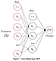Image source: Scikit Learn

## Implementation

Training the MLP classifier model (reference library) on the training set:

`from sklearn.neural_network import MLPClassifiermlp = MLPClassifier(alpha=1, max_iter=1000)mlp.fit(X_train, y_train)`

Predicting the test set results:

`y_pred_mlp = mlp.predict(X_test)`

Making the confusion matrix & calculating accuracy score:

`from sklearn.metrics import confusion_matrix, accuracy_scorecm = confusion_matrix(y_test, y_pred_mlp)print (cm)acc_mlp = accuracy_score(y_test, y_pred_mlp)print (acc_mlp)`

# Conclusion

Scikit Learn is a single-node framework and contains various effective tools for predictive data analysis. This works well for limited data. In case we need to work with massive amount of data, we can try Apache Spark MLlib.

Thanks for reading!! If you have enjoyed, Clap & Share it!! To see similar posts, follow me on Medium & LinkedIn.

Tech enthusiast, Azure Big Data Architect.

## More from Prosenjit Chakraborty

Tech enthusiast, Azure Big Data Architect.